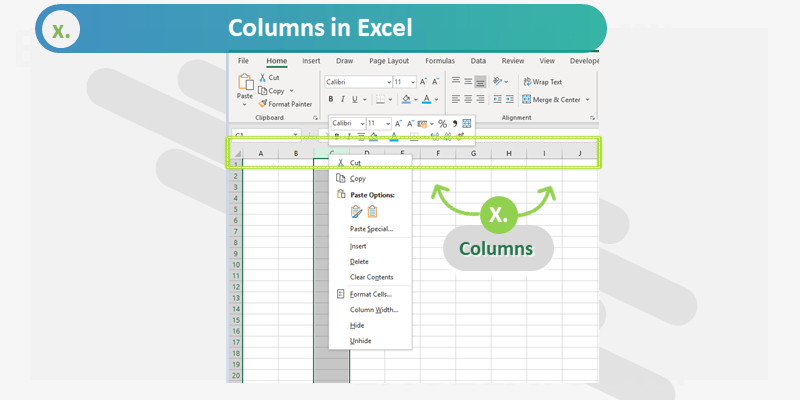Columns are Fields in Excel Spreadsheet. Excel Spreadsheet is container in the Workbook, it is in Tabular Format with Rows (Horizontal records) and Columns (Vertical fields). Each Column in a sheet represented with a unique Column Name, starts with Column name A.

### Limitations:

Many Excel users raises these common question a about Excel Columns. How many columns in Excel Worksheet? Is there any limitation with Columns in Excel?

There are 16,384 Columns in the latest version of Excel Application (from Office 2007 and higher).

The maximum width of an Excel Column can set to 255 Characters.

MS Office Version Row Limitation
Excel 2003 256
Excel 2007 16,384
Excel 2010 16,384
Excel 2013 16,384
Excel 2016 16,384
Excel 2019 16,384

### Usage & Properties

Here are most common uses of Excel Columns in our daily Excel tasks. We can do the following tasks and change the values of below Column properties if required.

Properties Usage
Width We can increase and decrease the Column width based on our requirement
Fill Color We can change the Interior Color if the Entire Column
Fonts We can select different font to a Column and format the font size, font name, font color, etc.
Hide/Unhide We can hide or unhide the Columns if required
Group We can Group Specific number of Columns in Excel to Expand and Collapse.
Clear We can Clear Entire Column (range of Columns) at a time
Insert We can Insert columns before or after a specif column
Delete We can delete any specific column if required

### Functions

Excel provided verity of built-in functions to deal with Columns. Here are the most commonly used Column Functions and Formulas.

Properties Usage
Column(address) Returns the Column number of the Given Address. Example: Row(D25) returns 4
Column() Returns the Column number of the Cell.
Previous Column Column()-1, return the previous Column of the Cell where the function is inserted. For example, if you insert this formula at J5, it returns 9.
Next Column Column()+1, return the next Column of the Cell where the function is inserted. For example, if you insert this formula at J5, it returns 11.
Columns() Returns the Column Numbers
Column(Range) Returns the Number of Column in a given Range. For example, =COLUMNS(A1:J2) returns 10.
Columns(1:1) Select the Entire first Column. For example, Sum(Column(5:5)) returns total of Column 5 values.
Absolute  Reference We can use \$ symbol to make a Column reference absolute. For example \$D5